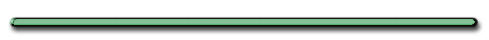HomeAP Physics

Conservation of Momentum

Elastic Collision?

No energy lost in collision

(1/2)m1v1+ (1/2)m2v2

= (1/2)m1v1f+ (1/2)m2v2f2

Combine equations above with

m1v1 + m2v2 = m1v1′ + m2v2

you get .....

V1 - V2 = V2' - V1'

Giancoli - page 189) 23

 m1 = .450 kg m1 = .450 kg V1 = 3.00 m/s V1' = m2 = .220 kg m2 = .900 kg V2 = 0 V2' =

V1 - V2 = V2 - V1

V2 - V1 = 3 m/s

V2 = 3 m/s + V1

Total Mom before = Total Mom After

 m1 = .450 kg m1 = .450 kg V1 = 3.00 m/s V1' = m2 = .900 kg m2 = .900 kg V2 = 0 V2' =

Total Mom before = Total Mom After

1.35 kgm/s = .45V1 + .90kg(3+V1)

1.35 = 1.35V1' + 2.7

V1'= -1 m/s or 1 m/s West

Since

V2 = 3 m/s + V1

then ...

V2 = 2 m/s East

Collisions in 2 Dimensions

Momentum is conserved
in x and y directions

Total Mom in X direction before =

Total Mom in X direction after

Total Mom in Y direction before =

Total Mom in Y direction after

If angle between = 90 degree ...

a2 + b2 = c2

Textbook - p. 190) 41

Total Momentum Before

a2 + b2 = c2

Eagle 1:

mv = 4.3 kg(7.8m/s) = 34 kgm/s

Eagle 2:

mv = 5.6kg(10.2 m/s) = 57kgm/s

Total Mom before:

a2 + b2 = c2

342 + 572 = c2

Total mom before = 66 kgm/s

66 kgm/s = (5.6kg + 4.3kg)v

V = 6.7 m/s

problem 42)

Gravity, Friction MomentumEnergyEnrichmentRifle Recoil
Dr. Dave's Hall of Fame